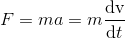## Can we assume that the mass of one object does not change?

Newton's second law of motion,can also be expressed asOnly when mass is a constant, the mass can be extracted out and the two expressions are equivalent.

I have to admit that I do not know if mass is constant or not. The mass is taken as constant in this article, is based on the following thought.

In algebra, one equation can be used to solve only one variable. If two variables are in it, any result will be arbitrary. Let's count how many variables are there in Newton's second law of motion. The F can be left out, because a fair comparison can not be done if nothing is common in two experiments. If among the m, v (or length), and t , all three of them are variables, then we need three equations. If two of them are variables, then two equations are needed. If only v is the variable, then one equation is enough.

In Special Relativity, one equation is used to solve two variables: v and t . If we take mass as another variable, how much randomness do we have to put in the solution?

In the first part of his paper, Einstein treated mass as a constant, else the mass would have appeared somewhere in the various steps leading to the theory of relativity. But in the last part of the paper, mass became changeable. So, this added another self-contradiction to this paper.

But this is nothing more than the misunderstanding of the principle of relativity, plus an arbitrary solution. That's why we had left it alone in the main article.